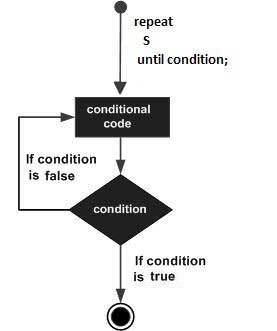# Pascal - Repeat-Until Loop

#### Learn Pascal Programming from Scratch

94 Lectures 8.5 hours

Unlike for and while loops, which test the loop condition at the top of the loop, the repeat ... until loop in Pascal checks its condition at the bottom of the loop.

A repeat ... until loop is similar to a while loop, except that a repeat ... until loop is guaranteed to execute at least one time.

## Syntax

```repeat
S1;
S2;
...
...
Sn;
until condition;
```

For example,

```repeat
sum := sum + number;
number := number - 2;
until number = 0;
```

Notice that the conditional expression appears at the end of the loop, so the statement(s) in the loop execute once before the condition is tested.

If the condition is false, the flow of control jumps back up to repeat, and the statement(s) in the loop execute again. This process repeats until the given condition becomes true.

## Flow Diagram### Example

```program repeatUntilLoop;
var
a: integer;

begin
a := 10;
(* repeat until loop execution *)
repeat
writeln('value of a: ', a);
a := a + 1
until a = 20;
end.
```

When the above code is compiled and executed, it produces the following result −

```value of a: 10
value of a: 11
value of a: 12
value of a: 13
value of a: 14
value of a: 15
value of a: 16
value of a: 17
value of a: 18
value of a: 19
```
pascal_loops.htm